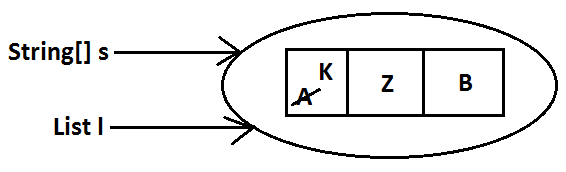# Collection Framework

Agenda

## Introduction:

1. An array is an indexed collection of fixed no of homogeneous data elements. (or)
2. An array represents a group of elements of same data type.
3. The main advantage of array is we can represent huge no of elements by using single variable. So that readability of the code will be improved.

### Limitations of Object[] array:

1. Arrays are fixed in size that is once we created an array there is no chance of increasing (or) decreasing the size based on our requirement hence to use arrays concept compulsory we should know the size in advance which may not possible always.
2. Arrays can hold only homogeneous data elements.
Example:
```Student[] s=new Student;
s=new Student();//valid
s=new Customer();//invalid(compile time error)
```
Compile time error:
```Test.java:7: cannot find symbol
Symbol: class Customer
Location: class Test
s=new Customer();
```
3) But we can resolve this problem by using object type array(Object[]).
Example:
```Object[] o=new Object;
o=new Student();
o=new Customer();
```
4) Arrays concept is not implemented based on some data structure hence ready-made methods support we can't expert. For every requirement we have to write the code explicitly.

To overcome the above limitations we should go for collections concept.

1. Collections are growable in nature that is based on our requirement we can increase (or) decrease the size hence memory point of view collections concept is recommended to use.
2. Collections can hold both homogeneous and heterogeneous objects.
3. Every collection class is implemented based on some standard data structure hence for every requirement ready-made method support is available being a programmer we can use these methods directly without writing the functionality on our own.

### Differences between Arrays and Collections ?

Arrays Collections
1) Arrays are fixed in size. 1) Collections are growable in nature.
2) Memory point of view arrays are not recommended to use. 2) Memory point of view collections are highly recommended to use.
3) Performance point of view arrays are recommended to use. 3) Performance point of view collections are not recommended to use.
4) Arrays can hold only homogeneous data type elements. 4) Collections can hold both homogeneous and heterogeneous elements.
5) There is no underlying data structure for arrays and hence there is no readymade method support. 5) Every collection class is implemented based on some standard data structure and hence readymade method support is available.
6) Arrays can hold both primitives and object types. 6) Collections can hold only objects but not primitives.

## Collection:

If we want to represent a group of objects as single entity then we should go for collections.

## Collection framework:

It defines several classes and interfaces to represent a group of objects as a single entity.
Java C++
Collection Containers
Collection framework STL(Standard Template Library)

## 9(Nine) key interfaces of collection framework:

1. Collection
2. List
3. Set
4. SortedSet
5. NavigableSet
6. Queue
7. Map
8. SortedMap
9. NavigableMap

### Collection:

1. If we want to represent a group of "individual objects" as a single entity then we should go for collection.
2. In general we can consider collection as root interface of entire collection framework.
3. Collection interface defines the most common methods which can be applicable for any collection object.
4. There is no concrete class which implements Collection interface directly.

### List:

1. It is the child interface of Collection.
2. If we want to represent a group of individual objects as a single entity where "duplicates are allow and insertion order must be preserved" then we should go for List interface.
Diagram:Vector and Stack classes are re-engineered in 1.2 versions to implement List interface.

### Set:

1. It is the child interface of Collection.
2. If we want to represent a group of individual objects as single entity "where duplicates are not allow and insertion order is not preserved" then we should go for Set interface.
Diagram:### SortedSet:

1. It is the child interface of Set.
2. If we want to represent a group of individual objects as single entity "where duplicates are not allow but all objects will be insertion according to some sorting order then we should go for SortedSet.
(or)
3. If we want to represent a group of "unique objects" according to some sorting order then we should go for SortedSet.

### NavigableSet:

1. It is the child interface of SortedSet.
2. It provides several methods for navigation purposes.

### Queue:

1. It is the child interface of Collection.
2. If we want to represent a group of individual objects prior to processing then we should go for queue concept.
Diagram:Note: All the above interfaces (Collection, List, Set, SortedSet, NavigableSet, and Queue) meant for representing a group of individual objects.
If we want to represent a group of objects as key-value pairs then we should go for Map.

### Map:

1. Map is not child interface of Collection.
2. If we want to represent a group of objects as key-value pairs then we should go for Map interface.
3. Duplicate keys are not allowed but values can be duplicated.
Diagram: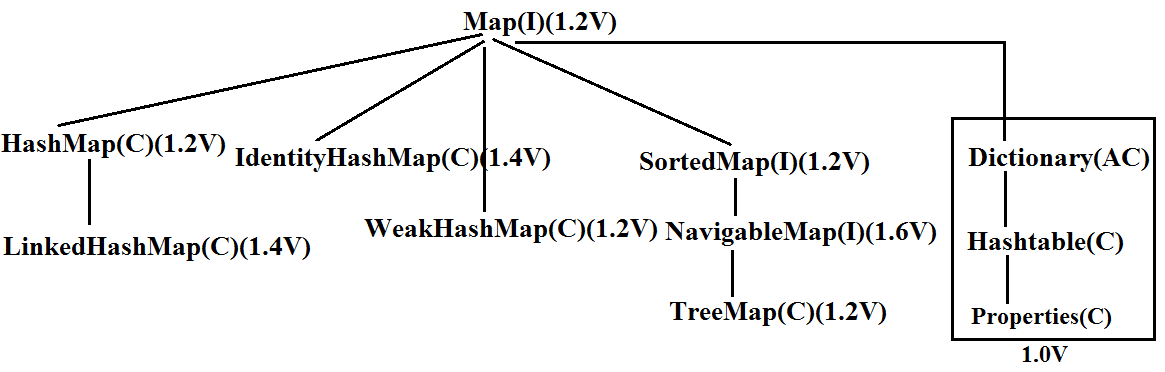### SortedMap:

1. It is the child interface of Map.
2. If we want to represent a group of objects as key value pairs "according to some sorting order of keys" then we should go for SortedMap.

### NavigableMap:

1) It is the child interface of SortedMap and defines several methods for navigation purposes.

#### What is the difference between Collection and Collections ?

"Collection is an "interface" which can be used to represent a group of objects as a single entity. Whereas "Collections is an utility class" present in java.util package to define several utility methods for Collection objects.

Collection--------------------interface
Collections------------------class

#### In collection framework the following are legacy characters.

1. Enumeration(I)
2. Dictionary(AC)
3. Vector(C)
4. Stack(C)
5. Hashtable(C)
6. Properties(C)
Diagram:Diagram:### Collection interface:

• If we want to represent a group of individual objects as a single entity then we should go for Collection interface. This interface defines the most common general methods which can be applicable for any Collection object.
• The following is the list of methods present in Collection interface.
3. boolean remove(Object o);
4. boolean removeAll(Object o);
5. boolean retainAll(Collection c);
To remove all objects except those present in c.
6. Void clear();
7. boolean contains(Object o);
8. boolean containsAll(Collection c);
9. boolean isEmpty();
10. Int size();
11. Object[] toArray();
12. Iterator iterator();
There is no concrete class which implements Collection interface directly.

### List interface:

• It is the child interface of Collection.
• If we want to represent a group of individual objects as a single entity where duplicates are allow and insertion order is preserved. Then we should go for List.
• We can differentiate duplicate objects and we can maintain insertion order by means of index hence "index play very important role in List".
List interface defines the following specific methods.
3. Object get(int index);
4. Object remove(int index);
5. Object set(int index,Object new);//to replace
6. Int indexOf(Object o);
Returns index of first occurrence of "o".
7. Int lastIndexOf(Object o);
8. ListIterator listIterator();

## ArrayList:

1. The underlying data structure is resizable array (or) growable array.
2. Duplicate objects are allowed.
3. Insertion order preserved.
4. Heterogeneous objects are allowed.(except TreeSet , TreeMap every where heterogenious objects are allowed)
5. Null insertion is possible.

### Constructors:

1) ArrayList a=new ArrayList();

Creates an empty ArrayList object with default initial capacity "10" if ArrayList reaches its max capacity then a new ArrayList object will be created with

New capacity=(current capacity*3/2)+1

2) ArrayList a=new ArrayList(int initialcapacity);

Creates an empty ArrayList object with the specified initial capacity.

3) ArrayList a=new ArrayList(collection c);

Creates an equivalent ArrayList object for the given Collection that is this constructor meant for inter conversation between collection objects. That is to dance between collection objects.

Demo program for ArrayList:
```import java.util.*;
class ArrayListDemo
{
public static void main(String[] args)
{
ArrayList a=new ArrayList();
System.out.println(a);//[A, 10, A, null]
a.remove(2);
System.out.println(a);//[A, 10, null]
System.out.println(a);//[A, 10, m, null, n]
}
}
```
• Usually we can use collection to hold and transfer objects from one tier to another tier. To provide support for this requirement every Collection class already implements Serializable and Cloneable interfaces.
• ArrayList and Vector classes implements RandomAccess interface so that any random element we can access with the same speed. Hence ArrayList is the best choice of "retrival operation".
• RandomAccess interface present in util package and doesn't contain any methods. It is a marker interface.

#### Example :

```ArrayList a1=new ArrayList();

System.out.println(a1 instanceof Serializable ); //true
System.out.println(a2 instanceof Clonable); //true

System.out.println(a1 instanceof RandomAccess); //true
System.out.println(a2 instanceof RandomAccess); //false

```

#### Differences between ArrayList and Vector ?

ArrayList Vector
1) No method is synchronized 1) Every method is synchronized
2) At a time multiple Threads are allow to operate on ArrayList object and hence ArrayList object is not Thread safe. 2) At a time only one Thread is allow to operate on Vector object and hence Vector object is Thread safe.
3) Relatively performance is high because Threads are not required to wait. 3) Relatively performance is low because Threads are required to wait.
4) It is non legacy and introduced in 1.2v 4) It is legacy and introduced in 1.0v

### Getting synchronized version of ArrayList object:

• Collections class defines the following method to return synchronized version of List.
Public static List synchronizedList(list l);
Example: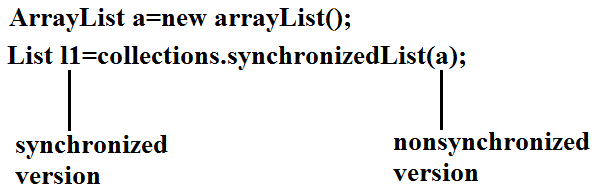• Similarly we can get synchronized version of Set and Map objects by using the following methods.
1) public static Set synchronizedSet(Set s);
2) public static Map synchronizedMap(Map m);
• ArrayList is the best choice if our frequent operation is retrieval.
• ArrayList is the worst choice if our frequent operation is insertion (or) deletion in the middle because it requires several internal shift operations.
Diagram: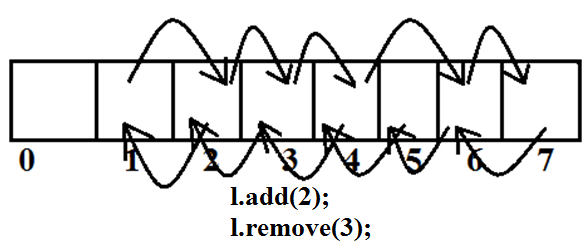1. The underlying data structure is double LinkedList
2. If our frequent operation is insertion (or) deletion in the middle then LinkedList is the best choice.
3. If our frequent operation is retrieval operation then LinkedList is worst choice.
4. Duplicate objects are allowed.
5. Insertion order is preserved.
6. Heterogeneous objects are allowed.
7. Null insertion is possible.
8. Implements Serializable and Cloneable interfaces but not RandomAccess.
Diagram:Usually we can use LinkedList to implement Stacks and Queues.
To provide support for this requirement LinkedList class defines the following 6 specific methods.

3. Object getFirst();
4. Object getLast();
5. Object removeFirst();
6. Object removeLast();
We can apply these methods only on LinkedList object.

### Constructors:

To create an equivalent LinkedList object for the given collection.
Example:
```import java.util.*;
{
public static void main(String[] args)
{
System.out.println(l);//[ashok, 30, null, ashok]
l.set(0,"software");
System.out.println(l);//[software, 30, null, ashok]
l.set(0,"venky");
System.out.println(l);//[venky, 30, null, ashok]
l.removeLast();
System.out.println(l);//[venky, 30, null]
System.out.println(l);//[vvv, venky, 30, null]
}
}
```

### Vector:

1. The underlying data structure is resizable array (or) growable array.
2. Duplicate objects are allowed.
3. Insertion order is preserved.
4. Heterogeneous objects are allowed.
5. Null insertion is possible.
6. Implements Serializable, Cloneable and RandomAccess interfaces.
Every method present in Vector is synchronized and hence Vector is Thread safe.

### Vector specific methods:

To remove elements:
1. remove(Object o);--------Collection
2. remove(int index);--------------List
3. removeElement(Object o);----Vector
4. removeElementAt(int index);-----Vector
5. removeAllElements();-----Vector
6. clear();-------Collection
To get objects:
1. Object get(int index);---------------List
2. Object elementAt(int index);-----Vector
3. Object firstElement();--------------Vector
4. Object lastElement();---------------Vector
Other methods:
1. Int size();//How many objects are added
2. Int capacity();//Total capacity
3. Enumeration elements();
Constructors:
1. Vector v=new Vector();
• Creates an empty Vector object with default initial capacity 10.
• Once Vector reaches its maximum capacity then a new Vector object will be created with double capacity. That is "newcapacity=currentcapacity*2".
2. Vector v=new Vector(int initialcapacity);
3. Vector v=new Vector(int initialcapacity, int incrementalcapacity);
4. Vector v=new Vector(Collection c);
Example:
```import java.util.*;
class VectorDemo
{
public static void main(String[] args)
{
Vector v=new Vector();
System.out.println(v.capacity());//10
for(int i=1;i<=10;i++)
{
}
System.out.println(v.capacity());//10
System.out.println(v.capacity());//20
System.out.println(v);//[1, 2, 3, 4, 5, 6, 7, 8, 9, 10, A]
}
}
```

### Stack:

1. It is the child class of Vector.
2. Whenever last in first out(LIFO) order required then we should go for Stack.

### Constructor:

It contains only one constructor.

Stack s= new Stack();

### Methods:

1. Object push(Object o);
To insert an object into the stack.
2. Object pop();
3. Object peek();
4. boolean empty();
Returns true if Stack is empty.
5. Int search(Object o);
Returns offset if the element is available otherwise returns "-1"
Example:
```import java.util.*;
class StackDemo
{
public static void main(String[] args)
{
Stack s=new Stack();
s.push("A");
s.push("B");
s.push("C");
System.out.println(s);//[A, B, C]
System.out.println(s.pop());//C
System.out.println(s);//[A, B]
System.out.println(s.peek());//B
System.out.println(s.search("A"));//2
System.out.println(s.search("Z"));//-1
System.out.println(s.empty());//false
}
}
```

### The 3 cursors of java:

If we want to get objects one by one from the collection then we should go for cursor. There are 3 types of cursors available in java. They are:
1. Enumeration
2. Iterator
3. ListIterator

### Enumeration:

1. We can use Enumeration to get objects one by one from the legacy collection objects.
2. We can create Enumeration object by using elements() method.
public Enumeration elements();
Enumeration e=v.elements();
using Vector Object
Enumeration interface defines the following two methods
1. public boolean hasMoreElements();
2. public Object nextElement();
Example:
```import java.util.*;
class  EnumerationDemo
{
public static void main(String[] args)
{
Vector v=new Vector();
for(int i=0;i<=10;i++)
{
}
System.out.println(v);//[0, 1, 2, 3, 4, 5, 6, 7, 8, 9, 10]
Enumeration e=v.elements();
while(e.hasMoreElements())
{
Integer i=(Integer)e.nextElement();
if(i%2==0)
System.out.println(i);//0 2 4 6 8 10
}
System.out.print(v);//[0, 1, 2, 3, 4, 5, 6, 7, 8, 9, 10]
}
}
```

### Limitations of Enumeration:

1. We can apply Enumeration concept only for legacy classes and it is not a universal cursor.
2. By using Enumeration we can get only read access and we can't perform remove operations.
3. To overcome these limitations sun people introduced Iterator concept in 1.2v.

### Iterator:

1. We can use Iterator to get objects one by one from any collection object.
2. We can apply Iterator concept for any collection object and it is a universal cursor.
3. While iterating the objects by Iterator we can perform both read and remove operations.

We can get Iterator object by using iterator() method of Collection interface.
public Iterator iterator();
Iterator itr=c.iterator();

Iterator interface defines the following 3 methods.
1. public boolean hasNext();
2. public object next();
3. public void remove();
Example:
```import java.util.*;
class IteratorDemo
{
public static void main(String[] args)
{
ArrayList a=new ArrayList();
for(int i=0;i<=10;i++)
{
}
System.out.println(a);//[0, 1, 2, 3, 4, 5, 6, 7, 8, 9, 10]
Iterator itr=a.iterator();
while(itr.hasNext())
{
Integer i=(Integer)itr.next();
if(i%2==0)
System.out.println(i);//0, 2, 4, 6, 8, 10
else
itr.remove();
}
System.out.println(a);//[0, 2, 4, 6, 8, 10]
}
}
```

### Limitations of Iterator:

1. Both enumeration and Iterator are single direction cursors only. That is we can always move only forward direction and we can't move to the backward direction.
2. While iterating by Iterator we can perform only read and remove operations and we can't perform replacement and addition of new objects.
3. To overcome these limitations sun people introduced listIterator concept.

### ListIterator:

1. ListIterator is the child interface of Iterator.
2. By using listIterator we can move either to the forward direction (or) to the backward direction that is it is a bi-directional cursor.
3. While iterating by listIterator we can perform replacement and addition of new objects in addition to read and remove operations

By using listIterator method we can create listIterator object.

public ListIterator listIterator();
ListIterator itr=l.listIterator();

(l is any List object)

ListIterator interface defines the following 9 methods.

1. public boolean hasNext();
2. public Object next(); forward
3. public int nextIndex();
4. public boolean hasPrevious();
5. public Object previous(); backward
6. public int previousIndex();
7. public void remove();
8. public void set(Object new);
Example:
```import java.util.*;
class  ListIteratorDemo
{
public static void main(String[] args)
{
System.out.println(l);//[balakrishna, venki, chiru, nag]
ListIterator itr=l.listIterator();
while(itr.hasNext())
{
String s=(String)itr.next();
if(s.equals("venki"))
{
itr.remove();
}
}
System.out.println(l);//[balakrishna, chiru, nag]
}
}
```
Case 1:
```if(s.equals("chiru"))
{
itr.set("chran");
}
Output:
[balakrishna, venki, chiru, nag]
[balakrishna, venki, chran, nag]
```
Case 2:
```if(s.equals("nag"))
{
}
Output:
[balakrishna, venki, chiru, nag]
[balakrishna, venki, chiru, nag, chitu]
```
The most powerful cursor is listIterator but its limitation is it is applicable only for "List objects".

### Compression of Enumeration Iterator and ListIterator ?

Property Enumeration Iterator ListIterator
1) Is it legacy ? Yes no no
2) It is applicable for ? Only legacy classes. Applicable for any collection object. Applicable for only list objects.
3) Moment? Single direction cursor(forward) Single direction cursor(forward) Bi-directional.
4) How to get it? By using elements() method. By using iterator()method. By using listIterator() method.
6) Methods hasMoreElement()
nextElement()
hasNext()
next()
remove()
9 methods.

### Set interface:

1. It is the child interface of Collection.
2. If we want to represent a group of individual objects as a single entity where duplicates are not allow and insertion order is not preserved then we should go for Set interface.
Diagram:Set interface does not contain any new method we have to use only Collection interface methods.

### HashSet:

1. The underlying data structure is Hashtable.
2. Insertion order is not preserved and it is based on hash code of the objects.
3. Duplicate objects are not allowed.
4. If we are trying to insert duplicate objects we won't get compile time error and runtime error add() method simply returns false.
5. Heterogeneous objects are allowed.
6. Null insertion is possible.(only once)
7. Implements Serializable and Cloneable interfaces but not RandomAccess.
8. HashSet is best suitable, if our frequent operation is "Search".

### Constructors:

1. HashSet h=new HashSet();
Creates an empty HashSet object with default initial capacity 16 and default fill ratio 0.75(fill ratio is also known as load factor).
2. HashSet h=new HashSet(int initialcapacity);
Creates an empty HashSet object with the specified initial capacity and default fill ratio 0.75.
3. HashSet h=new HashSet(int initialcapacity,float fillratio);
4. HashSet h=new HashSet(Collection c);
Note : After filling how much ratio new HashSet object will be created , The ratio is called "FillRatio" or "LoadFactor".

Example:
```import java.util.*;
class HashSetDemo
{
public static void main(String[] args)
{
HashSet h=new HashSet();
System.out.println(h);//[null, D, B, C, 10, Z]
}
}
```

1. It is the child class of HashSet.
2. LinkedHashSet is exactly same as HashSet except the following differences.
1) The underlying data structure is Hashtable. 1) The underlying data structure is a combination of LinkedList and Hashtable.
2) Insertion order is not preserved. 2) Insertion order is preserved.
3) Introduced in 1.2 v. 3) Introduced in 1.4v.
In the above program if we are replacing HashSet with LinkedHashSet the output is [B, C, D, Z, null, 10].That is insertion order is preserved.
Example:
```import java.util.*;
{
public static void main(String[] args)
{
System.out.println(h);//[B, C, D, Z, null, 10]
}
}
```

Note: LinkedHashSet and LinkedHashMap commonly used for implementing "cache applications" where insertion order must be preserved and duplicates are not allowed.

### SortedSet:

1. It is child interface of Set.
2. If we want to represent a group of "unique objects" where duplicates are not allowed and all objects must be inserting according to some sorting order then we should go for SortedSet interface.
3. That sorting order can be either default natural sorting (or) customized sorting order.

### SortedSet interface define the following 6 specific methods.

1. Object first();
2. Object last();
Returns the SortedSet whose elements are <obj.
4. SortedSet tailSet(Object obj);
It returns the SortedSet whose elements are >=obj.
5. SortedSet subset(Object o1,Object o2);
Returns the SortedSet whose elements are >=o1 but <o2.
6. Comparator comparator();
• Returns the Comparator object that describes underlying sorting technique.
• If we are following default natural sorting order then this method returns null.
Diagram: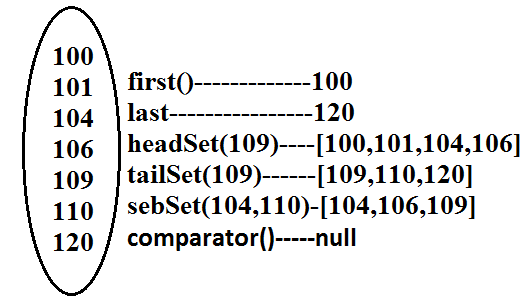## TreeSet:

1. The underlying data structure is balanced tree.
2. Duplicate objects are not allowed.
3. Insertion order is not preserved and it is based on some sorting order of objects.
4. Heterogeneous objects are not allowed if we are trying to insert heterogeneous objects then we will get ClassCastException.
5. Null insertion is possible(only once).

## Constructors:

1. TreeSet t=new TreeSet();
Creates an empty TreeSet object where all elements will be inserted according to default natural sorting order.
2. TreeSet t=new TreeSet(Comparator c);
Creates an empty TreeSet object where all objects will be inserted according to customized sorting order specified by Comparator object.
3. TreeSet t=new TreeSet(SortedSet s);
4. TreeSet t=new TreeSet(Collection c);
Example 1:
```import java.util.*;
class TreeSetDemo
{
public static void main(String[] args)
{
TreeSet t=new TreeSet();
System.out.println(t);//[A, B, L, Z, a]
}
}
```

### Null acceptance:

• For the empty TreeSet as the 1st element "null" insertion is possible but after inserting that null if we are trying to insert any other we will get NullPointerException.
• For the non empty TreeSet if we are trying to insert null then we will get NullPointerException.
Example 2:
```import java.util.*;
class TreeSetDemo
{
public static void main(String[] args)
{
TreeSet t=new TreeSet();
System.out.println(t);
}
}
Output:
Runtime Exception.
```
Note :
• Exception in thread "main" java.lang.ClassCastException: java.lang.StringBuffer cannot be cast to java.lang.Comparable
• If we are depending on default natural sorting order compulsory the objects should be homogeneous and Comparable otherwise we will get ClassCastException.
• An object is said to be Comparable if and only if the corresponding class implements Comparable interface.
• String class and all wrapper classes implements Comparable interface but StringBuffer class doesn't implement Comparable interface hence in the above program we are getting ClassCastException.

### Comparable interface:

Comparable interface present in java.lang package and contains only one method compareTo() method.
public int compareTo(Object obj);
Example:
obj1.compareTo(obj2);
Diagram: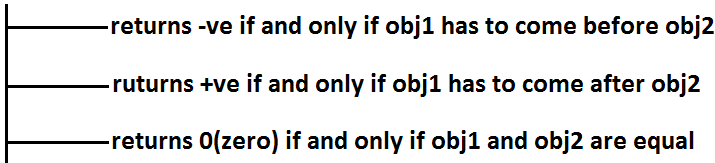Example 3:
```class Test
{
public static void main(String[] args)
{
System.out.println("A".compareTo("Z"));//-25
System.out.println("Z".compareTo("K"));//15
System.out.println("A".compareTo("A"));//0
//System.out.println("A".compareTo(new Integer(10)));
//Test.java:8: compareTo(java.lang.String) in java.lang.String cannot
be applied to (java.lang.Integer)
//System.out.println("A".compareTo(null));//NullPointerException
}
}
```

If we are depending on default natural sorting order then internally JVM will use compareTo() method to arrange objects in sorting order.

Example 4:
```import java.util.*;
class Test
{
public static void main(String[] args)
{
TreeSet t=new TreeSet();
System.out.println(t);//[0, 10, 15]
}
}
```

### compareTo() method analysis:• If we are not satisfying with default natural sorting order (or) if default natural sorting order is not available then we can define our own customized sorting by Comparator object.
• Comparable meant for default natural sorting order.
• Comparator meant for customized sorting order.

### Comparator interface:

Comparator interface present in java.util package this interface defines the following 2 methods.

#### 1) public int compare(Object obj1,Object Obj2);

Diagram:#### 2) public boolean equals(Object obj);

• Whenever we are implementing Comparator interface we have to provide implementation only for compare() method.
• Implementing equals() method is optional because it is already available from Object class through inheritance.

Requirement: Write a program to insert integer objects into the TreeSet where the sorting order is descending order.

```Program:
import java.util.*;
class Test
{
public static void main(String[] args)
{
TreeSet t=new TreeSet(new MyComparator());  //---->(1)
System.out.println(t);//[20, 15, 10, 5, 0]
}
}
class MyComparator implements Comparator
{
public int compare(Object obj1,Object obj2)
{
Integer i1=(Integer)obj1;
Integer i2=(Integer)obj2;
if(i1<i2)
return +1;
else if(i1 > i2)
return -100;
else return 0;
}
}
```
• At line "1" if we are not passing Comparator object then JVM will always calls compareTo() method which is meant for default natural sorting order(ascending order)hence in this case the output is [0, 5, 10, 15, 20].
• At line "1" if we are passing Comparator object then JVM calls compare() method of MyComparator class which is meant for customized sorting order(descending order) hence in this case the output is [20, 15, 10, 5, 0].
Diagram: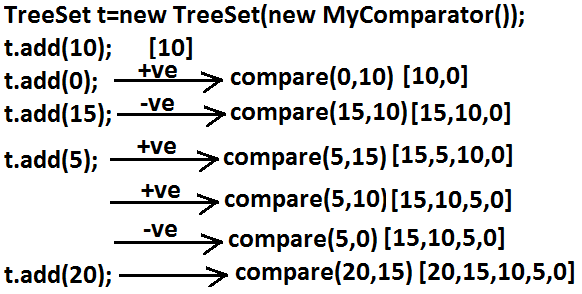```Various alternative implementations of compare() method:
public int compare(Object obj1,Object obj2)
{
Integer i1=(Integer)obj1;
Integer i2=(Integer)obj2;
//return i1.compareTo(i2);//[0, 5, 10, 15, 20]
//return -i1.compareTo(i2);//[20, 15, 10, 5, 0]
//return i2.compareTo(i1);//[20, 15, 10, 5, 0]
//return -i2.compareTo(i1);//[0, 5, 10, 15, 20]
//return -1;//[20, 5, 15, 0, 10]//reverse of insertion order
//return +1;//[10, 0, 15, 5, 20]//insertion order
//return 0;//and all the remaining elements treated as duplicate.
}
```
Requirement: Write a program to insert String objects into the TreeSet where the sorting order is reverse of alphabetical order.
```Program:
import java.util.*;
class TreeSetDemo
{
public static void main(String[] args)
{
TreeSet t=new TreeSet(new MyComparator());
System.out.println(t);//[ShobaRani, Roja, Ramulamma, RajaKumari, GangaBhavani]
}
}
class MyComparator implements Comparator
{
public int compare(Object obj1,Object obj2)
{
String s1=obj1.toString();
String s2=(String)obj2;
//return s2.compareTo(s1);
return -s1.compareTo(s2);
}
}
```
Requirement: Write a program to insert StringBuffer objects into the TreeSet where the sorting order is alphabetical order.
```Program:
import java.util.*;
class TreeSetDemo
{
public static void main(String[] args)
{
TreeSet t=new TreeSet(new MyComparator());
System.out.println(t);// [A, K, L, Z]
}
}
class MyComparator implements Comparator
{
public int compare(Object obj1,Object obj2)
{
String s1=obj1.toString();
String s2=obj2.toString();
return s1.compareTo(s2);
}
}
```
Note: Whenever we are defining our own customized sorting by Comparator then the objects need not be Comparable.
Example: StringBuffer
Requirement: Write a program to insert String and StringBuffer objects into the TreeSet where the sorting order is increasing length order. If 2 objects having the same length then consider they alphabetical order.
Program:
```import java.util.*;
class TreeSetDemo
{
public static void main(String[] args)
{
TreeSet t=new TreeSet(new MyComparator());
System.out.println(t);//[A, AA, xx, ABC, ABCD]
}
}
class MyComparator implements Comparator
{
public int compare(Object obj1,Object obj2)
{
String s1=obj1.toString();
String s2=obj2.toString();
int l1=s1.length();
int l2=s2.length();
if(l1 < l2)
return -1;
else if(l1 > l2)
return 1;
else
return s1.compareTo(s2);
}
}
```
Note: If we are depending on default natural sorting order then the objects should be "homogeneous and comparable" otherwise we will get ClassCastException. If we are defining our own sorting by Comparator then objects "need not be homogeneous and comparable".

### Comparable vs Comparator:

• For predefined Comparable classes default natural sorting order is already available if we are not satisfied with default natural sorting order then we can define our own customized sorting order by Comparator.
• For predefined non Comparable classes [like StringBuffer] default natural sorting order is not available we can define our own sorting order by using Comparator object.
• For our own classes [like Customer, Student, and Employee] we can define default natural sorting order by using Comparable interface. The person who is using our class, if he is not satisfied with default natural sorting order then he can define his own sorting order by using Comparator object.
Example:
```import java.util.*;
class Employee implements Comparable
{
String name;
int eid;
Employee(String name,int eid)
{
this.name=name;
this.eid=eid;
}
public String toString()
{
return name+"----"+eid;
}
public int compareTo(Object o)
{
int eid1=this.eid;
int eid2=((Employee)o).eid;
if(eid1 < eid2)
{
return -1;
}
else if(eid1 > eid2)
{
return 1;
}
else return 0;
}
}
class CompComp
{
public static void main(String[] args)
{
Employee e1=new Employee("nag",100);
Employee e2=new Employee("balaiah",200);
Employee e3=new Employee("chiru",50);
Employee e4=new Employee("venki",150);
Employee e5=new Employee("nag",100);
TreeSet t1=new TreeSet();
System.out.println(t1);//[chiru----50, nag----100, venki----150, balaiah----200]
TreeSet t2=new TreeSet(new MyComparator());
System.out.println(t2);//[balaiah----200, chiru----50, nag----100, venki----150]
}
}
class MyComparator implements Comparator
{
public int compare(Object obj1,Object obj2)
{
Employee e1=(Employee)obj1;
Employee e2=(Employee)obj2;
String s1=e1.name;
String s2=e2.name;
return s1.compareTo(s2);
}
}
```

### Compression of Comparable and Comparator ?

Comparable Comparator
1) Comparable meant for default natural sorting order. 1) Comparator meant for customized sorting order.
2) Present in java.lang package. 2) Present in java.util package.
3) Contains only one method.
compareTo() method.
3) Contains 2 methods.
Compare() method.
Equals() method.
4) String class and all wrapper Classes implements Comparable interface. 4) The only implemented classes of Comparator are Collator and RuleBasedCollator. (used in GUI)

### Compression of Set implemented class objects:

1) Underlying Data structure. Hashtable. LinkedList + Hashtable. Balanced Tree.
2) Insertion order. Not preserved. Preserved. Not preserved (by default).
3) Duplicate objects. Not allowed. Not allowed. Not allowed.
4) Sorting order. Not applicable. Not applicable. Applicable.
5) Heterogeneous objects. Allowed. Allowed. Not allowed.
6) Null insertion. Allowed. Allowed. For the empty TreeSet as the 1st element null insertion is possible in all other cases we will get NPE.

### Map:

Diagram:1. If we want to represent a group of objects as "key-value" pair then we should go for Map interface.
2. Both key and value are objects only.
3. Duplicate keys are not allowed but values can be duplicated
4. Each key-value pair is called "one entry".
Diagram: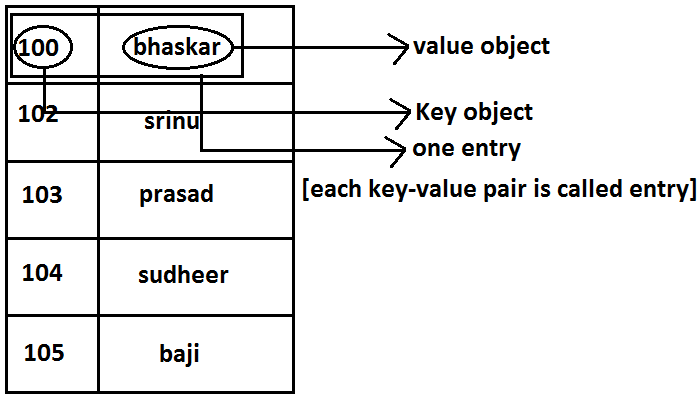• Map interface is not child interface of Collection and hence we can't apply Collection interface methods here.
• Map interface defines the following specific methods.
1. Object put(Object key,Object value);
To add an entry to the Map, if key is already available then the old value replaced with new value and old value will be returned.
Example:
```import java.util.*;
class Map
{
public static void main(String[] args)
{
HashMap m=new HashMap();
m.put("100","vijay");
System.out.println(m);//{100=vijay}
m.put("100","ashok");
System.out.println(m);//{100=ashok}
}
}
```
2. void putAll(Map m);
3. Object get(Object key);
4. Object remove(Object key);
It removes the entry associated with specified key and returns the corresponding value.
5. boolean containsKey(Object key);
6. boolean containsValue(Object value);
7. boolean isEmpty();
8. Int size();
9. void clear();
10. Set keySet();
The set of keys we are getting.
11. Collection values();
The set of values we are getting.
12. Set entrySet();
The set of entryset we are getting.

### Entry interface:

Each key-value pair is called one entry. Hence Map is considered as a group of entry Objects, without existing Map object there is no chance of existing entry object hence interface entry is define inside Map interface(inner interface).
Example:
```interface Map
{
.................;
.................;
.................;
interface Entry
{
Object getKey();
Object getValue();		    on Entry we can apply these 3 methods.
Object setValue(Object new);
}
}
```

### HashMap:

1. The underlying data structure is Hashtable.
2. Duplicate keys are not allowed but values can be duplicated.
3. Insertion order is not preserved and it is based on hash code of the keys.
4. Heterogeneous objects are allowed for both key and value.
5. Null is allowed for keys(only once) and for values(any number of times).
6. It is best suitable for Search operations.

### Differences between HashMap and Hashtable ?

HashMap Hashtable
1) No method is synchronized. 1) Every method is synchronized.
2) Multiple Threads can operate simultaneously on HashMap object and hence it is not Thread safe. 2) Multiple Threads can't operate simultaneously on Hashtable object and hence Hashtable object is Thread safe.
3) Relatively performance is high. 3) Relatively performance is low.
4) Null is allowed for both key and value. 4) Null is not allowed for both key and value otherwise we will get NullPointerException.
5) It is non legacy and introduced in 1.2v. 5) It is legacy and introduced in 1.0v.

### How to get synchronized version of HashMap:

By default HashMap object is not synchronized. But we can get synchronized version by using the following method of Collections class.
public static Map synchronizedMap(Map m1)

### Constructors:

1. HashMap m=new HashMap();
Creates an empty HashMap object with default initial capacity 16 and default fill ratio "0.75".
2. HashMap m=new HashMap(int initialcapacity);
3. HashMap m =new HashMap(int initialcapacity, float fillratio);
4. HashMap m=new HashMap(Map m);
Example:
```
import java.util.*;
class HashMapDemo
{
public static void main(String[] args)
{
HashMap m=new HashMap();
m.put("chiranjeevi",700);
m.put("balaiah",800);
m.put("venkatesh",200);
m.put("nagarjuna",500);
System.out.println(m);//{nagarjuna=500,venkatesh=200,balaiah=800,chiranjeevi=700}
System.out.println(m.put("chiranjeevi",100));//700
Set s=m.keySet();
System.out.println(s);//[nagarjuna,venkatesh,balaiah,chiranjeevi]
Collection c=m.values();
System.out.println(c);//[500, 200, 800, 100]
Set s1=m.entrySet();
System.out.println(s1);//[nagarjuna=500,venkatesh=200,balaiah=800,chiranjeevi=100]
Iterator itr=s1.iterator();
while(itr.hasNext())
{
Map.Entry m1=(Map.Entry)itr.next();
System.out.println(m1.getKey()+"......"+m1.getValue());
//nagarjuna......500	//venkatesh......200 //                            //balaiah......800 //chiranjeevi......100
if(m1.getKey().equals("nagarjuna"))
{
m1.setValue(1000);
}
}
System.out.println(m);                      //{nagarjuna=1000,venkatesh=200,balaiah=800,chiranjeevi=100}
}
}
```

It is exactly same as HashMap except the following differences :
1) The underlying data structure is Hashtable. 1) The underlying data structure is a combination of Hashtable+ LinkedList.
2) Insertion order is not preserved. 2) Insertion order is preserved.
3) introduced in 1.2.v. 3) Introduced in 1.4v.
Note: in the above program if we are replacing HashMap with LinkedHashMap then the output is {chiranjeevi=100, balaiah......800, venkatesh......200, nagarjuna......1000} that is insertion order is preserved.
Note: in general we can use LinkedHashSet and LinkedHashMap for implementing cache applications.

### IdentityHashMap:

It is exactly same as HashMap except the following differences:
1. In the case of HashMap JVM will always use ".equals()"method to identify duplicate keys, which is meant for content comparision.
2. But in the case of IdentityHashMap JVM will use== (double equal operator) to identify duplicate keys, which is meant for reference comparision.
Example:
```import java.util.*;
class HashMapDemo
{
public static void main(String[] args)
{
HashMap m=new HashMap();
Integer i1=new Integer(10);
Integer i2=new Integer(10);
m.put(i1,"pavan");
m.put(i2,"kalyan");
System.out.println(m);
}
}
```
• In the above program i1 and i2 are duplicate keys because i1.equals(i2) returns true.
• In the above program if we replace HashMap with IdentityHashMap then i1 and i2 are not duplicate keys because i1==i2 is false hence in this case the output is {10=pavan, 10=kalyan}.
```System.out.println(m.get(10));//null
10==i1------false
10==i2------false
```

### WeakHashMap:

It is exactly same as HashMap except the following differences:
• In the case of normal HashMap, an object is not eligible for GC even though it doesn't have any references if it is associated with HashMap. That is HashMap dominates garbage collector.
• But in the case of WeakHashMap if an object does not have any references then it's always eligible for GC even though it is associated with WeakHashMap that is garbage collector dominates WeakHashMap.
Example:
```import java.util.*;
class WeakHashMapDemo
{
public static void main(String[] args)throws Exception
{
WeakHashMap m=new WeakHashMap();
Temp t=new Temp();
m.put(t,"ashok");
System.out.println(m);//{Temp=ashok}
t=null;
System.gc();
System.out.println(m);//{}
}
}
class Temp
{
public String toString()
{
return "Temp";
}
public void finalize()
{
System.out.println("finalize() method called");
}
}
Output:
{Temp=ashok}
finalize() method called
{}
```
Diagram: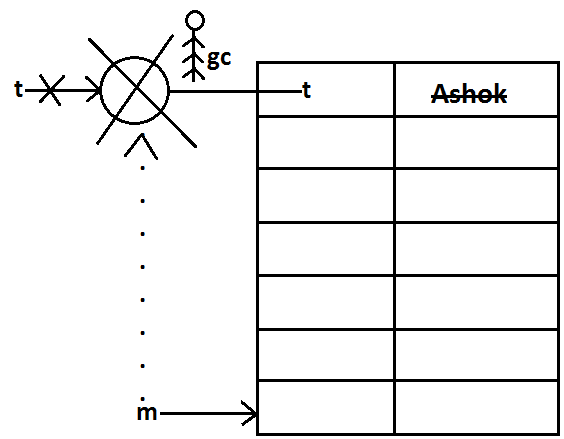In the above program if we replace WeakHashMap with normal HashMap then object won't be destroyed by the garbage collector in this the output is
```
{Temp=ashok}
{Temp=ashok}
```

### SortedMap:

• It is the child interface of Map.
• If we want to represent a group of key-value pairs according to some sorting order of keys then we should go for SortedMap.
• Sorting is possible only based on the keys but not based on values.
• SortedMap interface defines the following 6 specific methods.
1. Object firsyKey();
2. Object lastKey();
4. SortedMap tailMap(Object key);
5. SortedMap subMap(Object key1,Object key2);
6. Comparator comparator();

## TreeMap:

1. The underlying data structure is RED-BLACK Tree.
2. Duplicate keys are not allowed but values can be duplicated.
3. Insertion order is not preserved and all entries will be inserted according to some sorting order of keys.
4. If we are depending on default natural sorting order keys should be homogeneous and Comparable otherwise we will get ClassCastException.
5. If we are defining our own sorting order by Comparator then keys can be heterogeneous and non Comparable.
6. There are no restrictions on values they can be heterogeneous and non Comparable.
7. For the empty TreeMap as first entry null key is allowed but after inserting that entry if we are trying to insert any other entry we will get NullPointerException.
8. For the non empty TreeMap if we are trying to insert an entry with null key we will get NullPointerException.
9. There are no restrictions for null values.

### Constructors:

1. TreeMap t=new TreeMap();
For default natural sorting order.
2. TreeMap t=new TreeMap(Comparator c);
For customized sorting order.
3. TreeMap t=new TreeMap(SortedMap m);
4. TreeMap t=new TreeMap(Map m);
Example 1:
```import java.util.*;
class TreeMapDemo
{
public static void main(String[] args)
{
TreeMap t=new TreeMap();
t.put(100,"ZZZ");
t.put(103,"YYY");
t.put(101,"XXX");
t.put(104,106);
t.put(107,null);
//t.put("FFF","XXX");//ClassCastException
//t.put(null,"xxx");//NullPointerException
System.out.println(t);//{100=ZZZ, 101=XXX, 103=YYY, 104=106, 107=null}
}
}
```
Example 2:
```import java.util.*;
class TreeMapDemo
{
public static void main(String[] args)
{
TreeMap t=new TreeMap(new MyComparator());
t.put("XXX",10);
t.put("AAA",20);
t.put("ZZZ",30);
t.put("LLL",40);
System.out.println(t);//{ZZZ=30, XXX=10, LLL=40, AAA=20}
}
}
class MyComparator implements Comparator
{
public int compare(Object obj1,Object obj2)
{
String s1=obj1.toString();
String s2=obj2.toString();
return s2.compareTo(s1);
}
}
```

### Hashtable:

1. The underlying data structure is Hashtable.
2. Insertion order is not preserved and it is based on hash code of the keys.
3. Heterogeneous objects are allowed for both keys and values.
4. Null key (or) null value is not allowed otherwise we will get NullPointerException.
5. Duplicate keys are allowed but values can be duplicated.
6. Every method present inside Hashtable is syncronized and hence Hashtable objet is Thread-safe.

### Constructors:

1. Hashtable h=new Hashtable();
Creates an empty Hashtable object with default initialcapacity 11 and default fill ratio 0.75.
2. Hashtable h=new Hashtable(int initialcapacity);
3. Hashtable h=new Hashtable(int initialcapacity,float fillratio);
4. Hashtable h=new Hashtable (Map m);
Example:
```import java.util.*;
class HashtableDemo
{
public static void main(String[] args)
{
Hashtable h=new Hashtable();
h.put(new Temp(5),"A");
h.put(new Temp(2),"B");
h.put(new Temp(6),"C");
h.put(new Temp(15),"D");
h.put(new Temp(23),"E");
h.put(new Temp(16),"F");
System.out.println(h);//{6=C, 16=F, 5=A, 15=D, 2=B, 23=E}
}
}
class Temp
{
int i;
Temp(int i)
{
this.i=i;
}
public int hashCode()
{
return i;
}
public String toString()
{
return i+"";
}
}
```
Diagram:Note: if we change hasCode() method of Temp class as follows.
```public int hashCode()
{
return i%9;
}
```
Then the output is {16=F, 15=D, 6=C, 23=E, 5=A, 2=B}.
Diagram: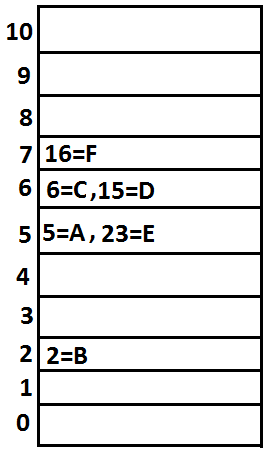Note: if we change initial capacity as 25.
Hashtable h=new Hashtable(25);
output is : { 23=E, 16=F, 15=D, 6=C, 5=A, 2=B }
Diagram:## Properties:

1. Properties class is the child class of Hashtable.
2. In our program if anything which changes frequently like DBUserName, Password etc., such type of values not recommended to hardcode in java application because for every change we have to recompile, rebuild and redeployed the application and even server restart also required sometimes it creates a big business impact to the client.
3. Such type of variable things we have to hardcode in property files and we have to read the values from the property files into java application.
4. The main advantage in this approach is if there is any change in property files automatically those changes will be available to java application just redeployment is enough.
5. By using Properties object we can read and hold properties from property files into java application.

### Constructor:

Properties p=new Properties();
In properties both key and value "should be String type only".

### Methods:

1. String getPrperty(String propertyname) ;
Returns the value associated with specified property.
2. String setproperty(String propertyname,String propertyvalue);
To set a new property.
3. Enumeration propertyNames();
4. void load(InputStream is);//Any InputStream we can pass.
To load Properties from property files into java Properties object.
5. void store(OutputStream os,String comment);//Any OutputStream we can pass.
To store the properties from Properties object into properties file.
Diagram:Example:
```import java.util.*;
import java.io.*;
class PropertiesDemo
{
public static void main(String[] args)throws Exception
{
Properties p=new Properties();
FileInputStream fis=new FileInputStream("abc.properties");
String s=p.getProperty("venki");
System.out.println(s);//8888
p.setProperty("nag","9999999");
Enumeration e=p.propertyNames();
while(e.hasMoreElements())
{
String s1=(String)e.nextElement();
System.out.println(s1);//nag
//user
//venki
}
FileOutputStream fos=new FileOutputStream("abc.properties");
p.store(fos,"updated by ashok for scjp demo class");
}
}
```
Property file: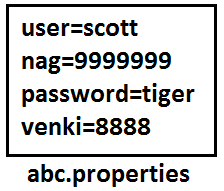Example:
```import java.util.*;
import java.io.*;
class PropertiesDemo
{
public static void main(String[] args)throws Exception
{
Properties p=new Properties();
FileInputStream fis=new FileInputStream("db.properties");
String url=p.getProperty("url");
String user=p.getProperty("user");
String pwd=p.getProperty("pwd");
Connection con=DriverManager.getConnection(url, user, pwd);
---------------------------------------------------------
--------------------------------------------------------
FileOutputStream fos=new FileOutputStream("db.properties");
p.store(fos,"updated by ashok for scjp demo class");
}
}
```

## 1.5 enhancements

### Queue interface

Diagram: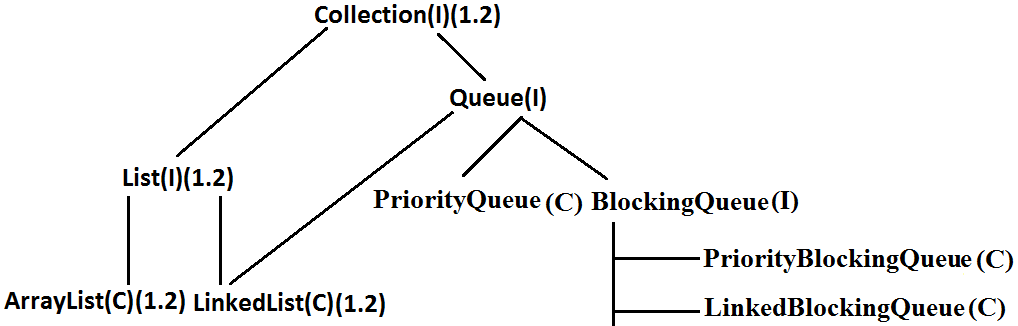1. Queue is child interface of Collections.
2. If we want to represent a group of individual objects prior (happening before something else) to processing then we should go for Queue interface.
3. Usually Queue follows first in first out(FIFO) order but based on our requirement we can implement our own order also.
4. From 1.5v onwards LinkedList also implements Queue interface.
5. LinkedList based implementation of Queue always follows first in first out order.

Assume we have to send sms for one lakh mobile numbers , before sending messages we have to store all mobile numbers into Queue so that for the first inserted number first message will be triggered(FIFO).

### Queue interface methods:

1. boolean affer(Object o);
To add an object to the Queue.
2. Object poll() ;
To remove and return head element of the Queue, if Queue is empty then we will get null.
3. Object remove();
To remove and return head element of the Queue. If Queue is empty then this method raises Runtime Exception saying NoSuchElementException.
4. Object peek();
To return head element of the Queue without removal, if Queue is empty this method returns null.
5. Object element();
It returns head element of the Queue and if Queue is empty then it will raise Runtime Exception saying NoSuchElementException.

### PriorityQueue:

1. PriorityQueue is a data structure to represent a group of individual objects prior to processing according to some priority.
2. The priority order can be either default natural sorting order (or) customized sorting order specified by Comparator object.
3. If we are depending on default natural sorting order then the objects must be homogeneous and Comparable otherwise we will get ClassCastException.
4. If we are defining our own customized sorting order by Comparator then the objects need not be homogeneous and Comparable.
5. Duplicate objects are not allowed.
6. Insertion order is not preserved but all objects will be inserted according to some priority.
7. Null is not allowed even as the 1st element for empty PriorityQueue.Otherwise we will get the "NullPointerException".

### Constructors:

1. PriorityQueue q=new PriorityQueue();
Creates an empty PriorityQueue with default initial capacity 11 and default natural sorting order.
2. PriorityQueue q=new PriorityQueue(int initialcapacity,Comparator c);
3. PriorityQueue q=new PriorityQueue(int initialcapacity);
4. PriorityQueue q=new PriorityQueue(Collection c);
5. PriorityQueue q=new PriorityQueue(SortedSet s);
Example 1:
```import java.util.*;
class PriorityQueueDemo
{
public static void main(String[] args)
{
PriorityQueue q=new PriorityQueue();
//System.out.println(q.peek());//null
//System.out.println(q.element());//NoSuchElementException
for(int i=0;i<=10;i++)
{
q.offer(i);
}
System.out.println(q);//[0, 1, 2, 3, 4, 5, 6, 7, 8, 9, 10]
System.out.println(q.poll());//0
System.out.println(q);//[1, 3, 2, 7, 4, 5, 6, 10, 8, 9]
}
}
```
Note: Some platforms may not provide proper supports for PriorityQueue [windowsXP].

Example 2:
```import java.util.*;
class PriorityQueueDemo
{
public static void main(String[] args)
{
PriorityQueue q=new PriorityQueue(15,new MyComparator());
q.offer("A");
q.offer("Z");
q.offer("L");
q.offer("B");
System.out.println(q);//[Z, B, L, A]
}
}
class MyComparator implements Comparator
{
public int compare(Object obj1,Object obj2)
{
String s1=(String)obj1;
String s2=obj2.toString();
return s2.compareTo(s1);
}
}
```

## 1.6v Enhancements :

### NavigableSet:

1. It is the child interface of SortedSet.
2. It provides several methods for navigation purposes.
Diagram: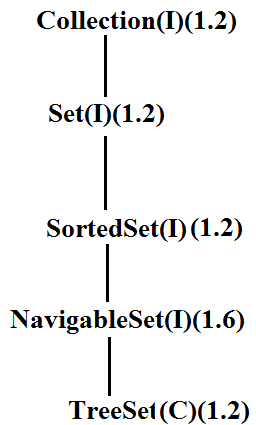NavigableSet interface defines the following methods.
1. ceiling(e);
It returns the lowest element which is >=e.
2. higher(e);
It returns the lowest element which is >e.
3. floor(e);
It returns highest element which is <=e.
4. lower(e);
It returns height element which is <e.
5. pollFirst ();
Remove and return 1st element.
6. pollLast ();
Remove and return last element.
7. descendingSet ();
Returns SortedSet in reverse order.
Diagram: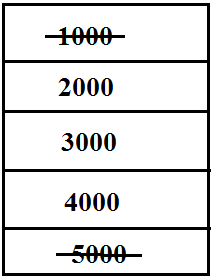Example:
```import java.util.*;
class NavigableSetDemo
{
public static void main(String[] args)
{
TreeSet<Integer> t=new TreeSet<Integer>();
System.out.println(t);//[1000, 2000, 3000, 4000, 5000]
System.out.println(t.ceiling(2000));//2000
System.out.println(t.higher(2000));//3000
System.out.println(t.floor(3000));//3000
System.out.println(t.lower(3000));//2000
System.out.println(t.pollFirst());//1000
System.out.println(t.pollLast());//5000
System.out.println(t.descendingSet());//[4000, 3000, 2000]
System.out.println(t);//[2000, 3000, 4000]
}
}
```

## NavigableMap:

It is the child interface of SortedMap and it defines several methods for navigation purpose.
Diagram: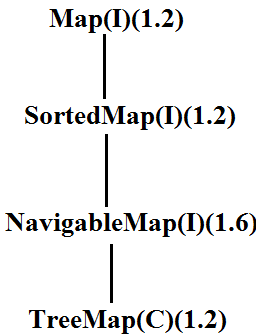NavigableMap interface defines the following methods.
1. ceilingKey(e);
2. higherKey(e);
3. floorKey(e);
4. lowerKey(e);
5. pollFirstEntry();
6. pollLastEntry();
7. descendingMap();
Example:
```import java.util.*;
class NavigableMapDemo
{
public static void main(String[] args)
{
TreeMap<String,String> t=new TreeMap<String,String>();
t.put("b","banana");
t.put("c","cat");
t.put("a","apple");
t.put("d","dog");
t.put("g","gun");
System.out.println(t);//{a=apple, b=banana, c=cat, d=dog, g=gun}
System.out.println(t.ceilingKey("c"));//c
System.out.println(t.higherKey("e"));//g
System.out.println(t.floorKey("e"));//d
System.out.println(t.lowerKey("e"));//d
System.out.println(t.pollFirstEntry());//a=apple
System.out.println(t.pollLastEntry());//g=gun
System.out.println(t.descendingMap());//{d=dog, c=cat, b=banana}
System.out.println(t);//{b=banana, c=cat, d=dog}
}
}
```
Diagram: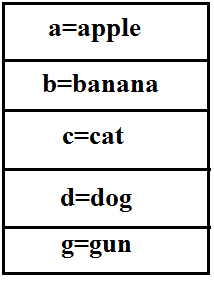## Collections class:

Collections class defines several utility methods for collection objects.

### Sorting the elements of a List:

Collections class defines the following methods to perform sorting the elements of a List.

public static void sort(List l);
• To sort the elements of List according to default natural sorting order in this case the elements should be homogeneous and comparable otherwise we will get ClassCastException.
• The List should not contain null otherwise we will get NullPointerException.
public static void sort(List l,Comparator c);
• To sort the elements of List according to customized sorting order.
Program 1: To sort elements of List according to natural sorting order.
```import java.util.*;
class CollectionsDemo
{
public static void main(String[] args)
{
ArrayList l=new ArrayList();
System.out.println("Before sorting :"+l);//[Z, A, K, N]
Collections.sort(l);
System.out.println("After sorting :"+l);//[A, K, N, Z]
}
}
```
Program 2: To sort elements of List according to customized sorting order.
```import java.util.*;
class CollectionsDemo
{
public static void main(String[] args)
{
ArrayList l=new ArrayList();
System.out.println("Before sorting :"+l);//[Z, A, K, L, 10]
Collections.sort(l,new MyComparator());
System.out.println("After sorting :"+l);//[Z, L, K, A, 10]
}
}
class MyComparator implements Comparator
{
public int compare(Object obj1,Object obj2)
{
String s1=(String)obj1;
String s2=obj2.toString();
return s2.compareTo(s1);
}
}
```
Collections class defines the following methods to search the elements of a List.
public static int binarySearch(List l,Object obj);
• If the List is sorted according to default natural sorting order then we have to use this method.
public static int binarySearch(List l,Object obj,Comparator c);
• If the List is sorted according to Comparator then we have to use this method.
Program 1: To search elements of List.
```import java.util.*;
class CollectionsSearchDemo
{
public static void main(String[] args)
{
ArrayList l=new ArrayList();
System.out.println(l);//[Z, A, M, K, a]
Collections.sort(l);
System.out.println(l);//[A, K, M, Z, a]
System.out.println(Collections.binarySearch(l,"Z"));//3
System.out.println(Collections.binarySearch(l,"J"));//-2
}
}
```
Diagram: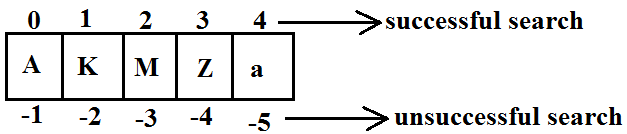Program 2:
```import java.util.*;
class CollectionsSearchDemo
{
public static void main(String[] args)
{
ArrayList l=new ArrayList();
System.out.println(l);//[15, 0, 20, 10, 5]
Collections.sort(l,new MyComparator());
System.out.println(l);//[20, 15, 10, 5, 0]
System.out.println(Collections.binarySearch(l,10,new MyComparator()));//2
System.out.println(Collections.binarySearch(l,13,new MyComparator()));//-3
System.out.println(Collections.binarySearch(l,17));//-6
}
}
class MyComparator implements Comparator
{
public int compare(Object obj1,Object obj2)
{
Integer i1=(Integer)obj1;
Integer i2=(Integer)obj2;
return i2.compareTo(i1);
}
}
```
Diagram:### Conclusions:

1. Internally these search methods will use binary search algorithm.
2. Successful search returns index unsuccessful search returns insertion point.
3. Insertion point is the location where we can place the element in the sorted list.
4. Before calling binarySearch() method compulsory the list should be sorted otherwise we will get unpredictable results.
5. If the list is sorted according to Comparator then at the time of search operation also we should pass the same Comparator object otherwise we will get unpredictable results.
Note:
For the list of n elements with respect to binary Search() method.
• Successful search range is: 0 to n-1.
• Unsuccessful search results range is: -(n+1)to -1.
• Total result range is: -(n+1)to n-1.
Example: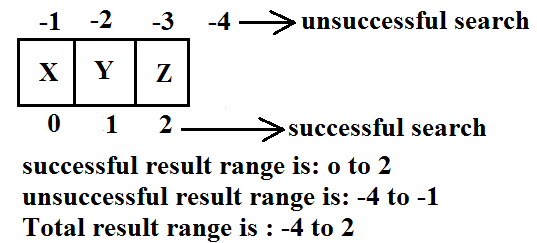Reversing the elements of List:
public static void reverse(List l);

reverse() vs reverseOrder() method
• We can use reverse() method to reverse the elements of List.
• Where as we can use reverseOrder() method to get reversed Comparator.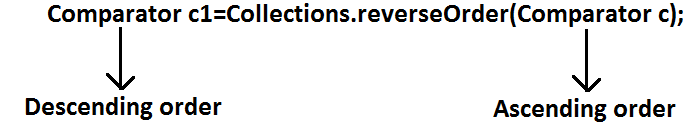Program: To reverse elements of list.
```import java.util.*;
class CollectionsReverseDemo
{
public static void main(String[] args)
{
ArrayList l=new ArrayList();
System.out.println(l);//[15, 0, 20, 10, 5]
Collections.reverse(l);
System.out.println(l);//[5, 10, 20, 0, 15]
}
}
```

## Arrays class:

Arrays class defines several utility methods for arrays.

### Sorting the elements of array:

• public static void sort(primitive[] p);//any primitive data type we can give
To sort the elements of primitive array according to default natural sorting order.
• public static void sort(object[] o);
To sort the elements of object[] array according to default natural sorting order.
In this case objects should be homogeneous and Comparable.
• public static void sort(object[] o,Comparator c);
To sort the elements of object[] array according to customized sorting order.
Note: We can sort object[] array either by default natural sorting order (or) customized sorting order but we can sort primitive arrays only by default natural sorting order.

Program: To sort elements of array.
```import java.util.*;
class ArraySortDemo
{
public static void main(String[] args)
{
int[] a={10,5,20,11,6};
System.out.println("primitive array before sorting");
for(int a1:a)
{
System.out.println(a1);
}
Arrays.sort(a);
System.out.println("primitive array after sorting");
for(int a1: a)
{
System.out.println(a1);
}
String[] s={"A","Z","B"};
System.out.println("Object array before sorting");
for(String s1: s)
{
System.out.println(s1);
}
Arrays.sort(s);
System.out.println("Object array after sorting");
for(String s1:s)
{
System.out.println(s1);
}
Arrays.sort(s,new MyComparator());
System.out.println("Object array after sorting by Comparator:");
for(String s1: s)
{
System.out.println(s1);
}
}
}
class MyComparator implements Comparator
{
public int compare(Object obj1,Object obj2)
{
String s1=obj1.toString();
String s2=obj2.toString();
return s2.compareTo(s1);
}
}
```

### Searching the elements of array:

Arrays class defines the following methods to search elements of array.
1. public static int binarySearch(primitive[] p,primitive key);
2. public static int binarySearch(Object[] p, object key);
3. public static int binarySearch(Object[] p,Object key,Comparator c);
All rules of Arrays class binarySearch() method are exactly same as Collections class binarySearch() method.

Program: To search elements of array.
```import java.util.*;
class ArraysSearchDemo
{
public static void main(String[] args)
{
int[] a={10,5,20,11,6};
Arrays.sort(a);
System.out.println(Arrays.binarySearch(a,6));//1
System.out.println(Arrays.binarySearch(a,14));//-5
String[] s={"A","Z","B"};
Arrays.sort(s);
System.out.println(Arrays.binarySearch(s,"Z"));//2
System.out.println(Arrays.binarySearch(s,"S"));//-3
Arrays.sort(s,new MyComparator());
System.out.println(Arrays.binarySearch(s,"Z",new MyComparator()));//0
System.out.println(Arrays.binarySearch(s,"S",new MyComparator()));//-2
System.out.println(Arrays.binarySearch(s,"N"));//-4(unpredictable result)
}
}
```

## Converting array to List:

Arrays class defines the following method to view array as List.
public static List asList (Object[] o);
• Strictly speaking we are not creating an independent List object just we are viewing array in List form.
• By using List reference if we are performing any change automatically these changes will be reflected to array reference similarly by using array reference if we are performing any change automatically these changes will be reflected to the List reference.
• By using List reference if we are trying to perform any operation which varies the size then we will get runtime exception saying UnsupportedOperationException.
• By using List reference if we are trying to insert heterogeneous objects we will get runtime exception saying ArrayStoreException.
Program: To view array in List form.
```import java.util.*;
class ArraysAsListDemo
{
public static void main(String[] args)
{
String[] s={"A","Z","B"};
List l=Arrays.asList(s);
System.out.println(l);//[A, Z, B]
s="K";
System.out.println(l);//[K, Z, B]
l.set(1,"L");
for(String s1: s)
System.out.println(s1);//K,L,B